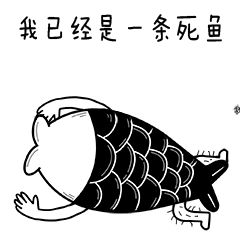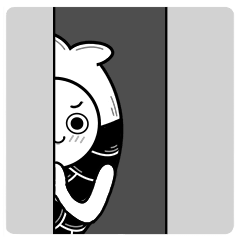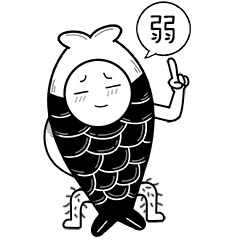• 流行歌曲
• 随便听听
• 经典歌曲
• 网络歌曲

• 懂得真爱

魏子熙

• 幻化成奇迹

魏子熙

• 戴上戒指

周燕

• 飞不过时间的爱

石惠文

• 完全放弃去爱你

石惠文

• 安静一会

廖茂伊

• 别忘了加速

廖茂伊

• 爱情水杯

林登科

• 丢失的吻

廖茂伊

• 说不出的甜美

高红霞

• 把你手儿牵

林登科

• 噩梦哭醒了

廖茂伊

• 爱不是种占有

魏子熙

• 不安常在

石惠文

• 单纯的逃避

周燕

• 别让爱失去力气

廖茂伊

• 北纬45度

韩宗言

• 查看你的简讯

毕金玲

• 还记得吗

曲秋红

• 某年某月某日

曲秋红

• 月亮和爱人

廉广

• 别再伤神

石惠文

• 不能忘却

李亮

• 寂寞夜里落泪

魏子熙

• 春风都在难过

毕金玲

• 无悔的赌注

曹泽林

• 撑起一片天

魏子熙

• 就像回到昨天

曹泽林

• 大鸭梨

周燕

• 对不起我爱你

曲秋红

• 我们在一起

曹泽林

• 迟迟没有归期

周燕

• 嫦娥与我

韩宗言

• 带来的伤悲

周燕

• 当眼睛说出泪水

韩宗言

• 孤单的怀念

李亮

• 就算时间匆匆过

石惠文

• 纯白色的天空

魏子熙

• 爱情的暖阳

曹泽林

• 爱又何必盛开

曹泽林

• 为他受了折磨

高红霞

• 誓言都是玩笑

石惠文

• 未来约定

高红霞

• 孤独入睡

魏子熙

• 充满了负荷

周燕

• 触碰不到的感动

周燕

• 爱惯性离开

石惠文

• 双手相执

高红霞

• 叫人不放心

李亮

• 老朋友

李亮

• 被时间渐渐搁浅

韩宗言

• 等你拉开我的帷幕

周燕

• 定格在从前

廖茂伊

• 彼此的心

毕金玲

• 就这样吧

韩宗言

• 没有爱也一样

石惠文

• 飞翔吧我的爱人

曲秋红

• 带来那份惊喜

石惠文

• 背上这行囊

廖茂伊

• 单身也很好

石惠文

• 红尘醉

蔺晓宇

• 离合缘缺

李亮

• 到底要爱如何

石惠文

• 昨天都不能回头

曹泽林

• 大声的说爱我

韩宗言

• 别人的可怜

毕金玲

• 相思雨

高红霞

• 不知道他有她

周燕

• 把你心打开

魏子熙

• 不安感情游戏

廖茂伊

• 暧昧的眼神

林登科

• 被城市淹没

廖茂伊

• 感慨万千

魏子熙

• 让安分快出逃

高红霞

• 请不要难过

李亮

• 难以忘记的事情

曹泽林

• 快去追吧

韩宗言

• 给我一个理由

曲秋红

• 可以飞翔

曲秋红

• 矛盾体

曲秋红

• 迷失的勇气

李亮

• 风雨同舟

蔺晓宇

• 我曾经幼稚

曹泽林

• 美丽雪花

曲秋红

• 不够坚决

毕金玲

• 你像花一样存在

曹泽林

• 你会记得我么

李亮

• 若即若离的爱

廉广

• 剩下我自己

高红霞

• 残余的温柔

毕金玲

• 当缘来的时候

周燕

• 从来没相信爱情

韩宗言

• 滑天下之大稽

蔺晓宇

• 感动无痕

李亮

• 被你成天指责

毕金玲

• 单相思最累了

周燕

• 曾以为爱丢了

周燕

• HAPPY

廉广

• 寂寥的长街

魏子熙

• 不要让你去打扰

韩宗言

• 你不再回来了

曹泽林

• 你说

廉广

• 八啦啦

韩宗言

• 冰冷的不只是眼泪

廖茂伊

• 睁开双眼

廉广

• 街边童谣

李亮

• 单纯的快乐

魏子熙

• 打不通的电话

魏子熙

• 空荡荡的梦

韩宗言

• 都老了很多

魏子熙

• 窗上的雨点

周燕

• 让我们相恋

石惠文

• 但爱不能丢

周燕

• 沉醉在美梦

周燕

• 安静的嘶吼

韩宗言

• 隐藏的别样生活

曹泽林

• 陌生了许多

曲秋红

• 从朋友到恋人

毕金玲

• 弹琴唱歌

韩宗言

• 超级马力

韩宗言

• 没有缺陷

曲秋红

• 放肆天使

李亮

• 都是想象的美

石惠文

• 煎熬的岁月

李亮

• 道德都丢掉

周燕

• 把你让给他

廖茂伊

• 触碰了你的心

魏子熙

• 别再依赖我

魏子熙

• 吹不散孤独

周燕

• 沉默

曲秋红

• 华灯初上

蔺晓宇

• 飞翔的幸福

李亮

• 都是因为你

廖茂伊

• 不过相识一场

魏子熙

• 只剩下哭泣

曲秋红

• 被你冷落的我

魏子熙

• 无声燃烧

高红霞

• 我们的恋曲

高红霞

• 情难自禁

高红霞

• 沧海苍田

韩宗言

• 穿向人海中

周燕

• 宝贝你在听吗

韩宗言

• 不该再出现

廖茂伊

• 将要离开你

李亮

• 话不多说

蔺晓宇

• 化装舞会

蔺晓宇

• 爱靠什么维持

蔺晓宇

• 挥挥手不愿走

蔺晓宇

• 牵手还是分手

高红霞

• 爱的滋味

毕金玲

• 心中那份爱

曹泽林

• 感受到的悲伤

李亮

• 曾经那些爱情里

韩宗言

• 曾相约月下

魏子熙

• 从生活抽离

毕金玲

• 两个人的约定

韩宗言

• 守候在零点

石惠文

• 难忘旧模样

曹泽林

• 撑起一把伞

周燕

• 他的背影很帅

石惠文

• 安静的融化

毕金玲

• 安静的睡

林登科

• 多看你几眼

廖茂伊

• 猝然消逝

毕金玲

• 花好月圆

蔺晓宇

• 强求不来

高红霞

• 多想喝醉

蔺晓宇

• 青春的记忆

李亮

• 难忘曾经的岁月

曹泽林

• 到底该怎样

毕金玲

• 荡到谷底

毕金玲

• 不是你的情人

魏子熙

• 打开这扇窗

毕金玲

• 我不想再提

高红霞

• 不会在心酸

林登科

• 变的很伤感

毕金玲

• 你是否了解

高红霞

• 超负荷的感情

毕金玲

• 黑白电影

蔺晓宇

• 不眠不休

蔺晓宇

• 没有向前的方向

李亮

• 成全你的自私

毕金玲

• 爱还在不在

廖茂伊

• 百分百的感觉

韩宗言

• 爱情在掉眼泪

韩宗言

• 呼唤

蔺晓宇

• 不在内疚

曲秋红

• 背靠背数星星

毕金玲

• 濒临破碎了

毕金玲

• 和平分手

蔺晓宇

• 飞鸟

蔺晓宇

• 金色的秋天

李亮

• 爱的深处

曲秋红

• 代替你说分手

魏子熙

• 陌生的问候

李亮

• 多久没为你演奏

曲秋红

• 平行线

李亮

• 痴心的骗局

周燕

• 我自己流浪

高红霞

• 祭奠我的故事

曹泽林